• Submissions# Modelling Zero-inflated Bivariate Count Responses using Marginal-Conditional Approach with application to Traffic Accidents and Fatalities Data

Tariqul Hasan M*, Rafiqul I Chowdhury and Ataharul Islam M

Submission: September 30, 2019;Published: December 10, 2019

DOI: 10.31031/EAES.2019.06.000642ISSN 2578-0336
Volume6 Issue4

#### Abstract

Zero-inflated bivariate count responses frequently occur in medical, environmental, ecological, social and transportation studies. These bivariate count responses recorded from a group of independent subjects or from a specific time or place may suffer the presence of excessive zeros, so constitute complexity while modelling. Although the responses under the umbrella of zero-inflated clustered and longitudinal count setup have been studied extensively in the past two decades, the literature is rather limited for analyzing zero-heavy bivariate count data. In this paper, we propose a marginal-conditional approach based bivariate zero-inflated Poisson-Poisson regression model to overcome the complexity imposed due to excessive zeros in bivariate count responses. The estimation and test procedures are developed using the likelihood function evolved from the marginal and conditional approaches. The proposed approach will be illustrated with the application to two traffic accidents and fatalities data collected from Virginia, USA and Groningen, The Netherlands.

Keywords: Correlated data; Poisson-poisson; Likelihood approach; Zero-heavy; Generalized linear model

#### Introduction

The problem of the presence of excessive zero values in the response variables is one of the major concerns in modeling count data. This problem arises in various fields such as biosocial science Long et al. , ecological studies Wang et al. , fishery studies Arab et al.  and Smith et al.  health studies Neelon et al. & Morgan et al. and Kassahun et al. [5-7] traﬃc accidents studies Papageorgiou, Meintanis [8,9] and the references therein. This characteristic of the presence of excessive zeros in the count data poses considerable challenges while modelling such data. Another issue of concern is the overdispersion stemming from the count data if the variance of the count responses is greater than mean, which might be attributed by excess zeros in the data. Since the work of Lambert , several models have been proposed to take account of the zero inflation in the count data in various fields, however, the problem with bivariate zero inflated models have not been discussed extensively in the literature.

Our work is motivated by two traﬃc accidents data sets. In the first data set, the daily number of accidents and the corresponding number of fatalities were investigated by Virginia state police between January 1, 1969 and October 31, 1970 Leiter & Hamdan . In the second data set, the number of monthly accidents and corresponding fatalities were recorded between January 1997 and December 2004 in Groningen, The Netherlands Meintanis . In both of these data sets, the first response variable represents number of accidents occurred during a particular time period. As there may not have any occurrence of accidents in a given time period, the first response variable i.e. number of traﬃc accidents may suﬀer the presence of excessive zeros. The second variable represents the count responses of the number of fatalities at a given time period related to the traﬃc accidents at that specific time period.

If the response of the first variable is zero i.e. no traﬃc accident occurs, the response of the second variable i.e. number of fatalities will also be zero. However, if the response of the first variable is non-zero, the response of the second variable still may be zero as the occurrence of accidents do not necessarily mean the occurrence of fatalities. Thus, the outcomes of the second response variable are conditionally related to the outcome of the first response variable. To model this type of data, one may require a modelling technique using an extended generalized linear model approach on the basis of marginal and conditional distributions to express the joint distribution of the Poisson outcomes for both the marginal and conditional variables. The use of the generalized linear model in this context makes the inferential procedures easier.

The bivariate Poisson regression models have been developed to address an increasingly important area of research with wide range of applications [12-15] in various fields where paired count data are correlated. Leiter & Hamdan  suggested bivariate probability models such as Poisson-binomial and Poisson-Poisson, with application to the traﬃc accidents and fatalities data. Following their approach, Cacoullos & Papageorgiou  proposed an alternative Poisson-binomial approach to model the relationship between the number of accidents and number of fatalities. Later Cacoullos & Papageor-giou  proposed a negative binomial-Poisson approach to analyze traﬃc accidents. The maximum likelihood approach-based parameter estimation techniques for negative binomial-Poisson models with their properties were proposed by Papageorgiou & Loukas . Meintanis  proposed goodness-of-fit test procedures applicable to traﬃc accident data. Several other studies also reviewed and examined bivariate Poisson distribution in the literature Holgate, Consul, Consul , Consul & Shoukri [18-21].

Based on the Poisson-Poisson distribution proposed by Leiter , a bivariate generalized model is developed by Islam & Chowdhury  where the link functions are obtained for the bivariate Poisson model and inferential procedures are shown. As the zero-inflated responses restrict the use of the above described modelling approaches available in the literature, a further improvement of the model is essential to take account of the excessive zeros in the bivariate count data. Univariate zero-inflated Poisson (ZIP) model was extended to bivariate ZIP by Li et al.  to model the mixture of bivariate Poisson distribution and a point mass at (0,0).

Their approach was further extended with the application to workplace injury data by Wang et al. A bivariate Poisson-Poisson regression model was proposed by Cheung & Lam  using the bivariate Poisson distribution by Holgate  applicable to bimodal distributions of counts with one mode at zero. Their bivariate Poisson-Poisson ZIP model was also extended under this constraint that Response 1 ≥ Response 2. But the setup of our data set is diﬀerent than the methodologies available in the literature. This is because, in the traﬃc accident data if Response 1 (number of accidents) is zero, then Response 2 (number of fatalities) has to be zero as well.

Thus, one needs to incorporate this constraint while modelling such data set. Consequently,  in this paper, we introduce a bivariate Poisson generalized linear model suitable for zero heavy count data with applications to traﬃc accident data. Our proposed approach is more general as it is based on the marginal and conditional distributions of two count variables where the marginal distribution of the first variable and the conditional distribution of the second variable for given value of the first variable follow Poisson distributions. The proposed model is expressed in exponential form and the link functions are obtained for generalized linear model of the bivariate Poisson outcomes and zero-inflation is taken into account for both the count outcomes. Although we illustrate the proposed approaches in two traﬃc accidents data sets, our approach is rather general and can be employed in other areas as well. For example, in health studies, the number of medical conditions diagnosed by the doctors for a group of patients and the number of health care facilities used by  that group   of patients may be an example of using a such modelling approach.

The plan of this paper is as follows. After introducing the bivariate zero-inflated Poisson model in Section 2, we provide the estimation techniques of the model parameters in Section 3. Application of the proposed model to the traﬃc accidents and fatalities data is presented in Section 4 and some concluding remarks are presented in the Section 5.

#### Bivariate Zero-Inflated Poisson-Poisson Model

In this section we introduce a marginal-conditional approach based bivariate zero-inflated Poisson-Poisson model for count data with excessive zeros. Let Yi1 be the count response recorded for the ith (i = 1, 2, …, n) day or month. For example, for the Virginia traffic accidents and fatalities data, the Yi1 represents number of daily accident counts recorded for the ith (i = 1, 2, … , n=639) day. Similarly, for the Groningen's traffic accidents and fatalities data, the Yi1 represents number of monthly accident counts recorded for the ith (i = 1, 2, … , n=96) month. The response variable may have excessive zeros as many responses may result zero counts due to the fact that there may not have any accident recorded in any given time point (either day or month according to our data examples).

We also assume that Yi2 represent the count responses of the number of fatalities occurred from the accidents of the ith time point. As mentioned earlier that if there is no accident recorded in any given time point (i.e. Yi1 = 0), then the analogous response for the second variable is recorded as zero i.e.  Yi2 = 0.  This is because no fatalities can occur if there is no accident. Moreover, occurrences of accidents do not necessarily mean the occurrences of fatalities. Thus, the proportion of zeros are likely to be higher for Yi2  as compared to Yi1.  Our approach is based on the following two assumptions.

Assumption #1: Let Yi1 follows a zero-inflated Poisson distribution with probability mass functionWhere Yi1* follows Poisson distribution with mean λ1i with. In (1),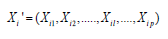represents the vector of P-dimensional covariates and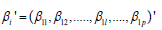represents the P-dimensional vector of regression parameters. The probability parameter 1 φ in (1) represents the proportion of excessive zeros in the count responses.

Based on assumption # 1, the probability mass function can be expressed asIt can be shown thatIf responses do not have any excessive zeroes (i.e. 1 φ = 0), then the probability mass function 1 ( ) i g Y in (2) is simplified to a Poisson distribution with rate 1i λ .

Assumption #2: Given Yi1 , let Yi2 , the random variable for the count of the second variable resulting from the ith subject, have the following conditional distribution asWhere λi2 = exp(X′iβ2) with Xi is as defined in (2) and β2 = (β21, β22, . . . , β2l, . . . , β2P)′ represents the P -dimensional vector of regression parameters on the response variable Yi2.

The above expression in (3) is developed based on the fact that Yi2 will have three diﬀerent categories. This is because as Yi1 = 0 with probability ϕ1, then Yi2 has to be 0 with probability ϕ1 as well. If Yi1 > 0 , then Yi2 still can be 0 with probability ϕ2 or Poisson distribution with mean λi2Yi1 with probability 1-ϕ1−ϕ2. Note that similar to Assumption 1, if the responses do not have excessive zeros (i.e. ϕ1=0 and ϕ2=0), then the conditional mass function in (3) reduces to a Poisson distribution with parameter λi2Yi1. Thus, the bivariate joint mass function can be achieved by multiplying the marginal and conditional mass function derived in (2) and (3). Therefore, the bivariate mass function for Yi1 and Yi2 can be expressed asUnder the assumption that the data do not have excessive zeros (i.e. φ1= 0 and φ2 = 0), the bivariate mass function in (4) will be simplied to the regular bivariate Poisson mass function which can be written as the bivariate exponential formThe bivariate zero-inflated Poisson mass function derived in (4) will be used to develop the likelihood function based estimating equation to estimate the model parameters.

Parameter estimation

In this section we present the estimation techniques of the model parameters based on likelihood approach. The likelihood function for the bivariate zero inflated Poisson model can be expressed asWhere 1 2 ( , ) i i δ Y Y is the delta function which is equal to 1 if the condition inside the parenthesis is simplified or 0 otherwise. To estimate the regression parameters, we use the likelihood estimating equation which was derived by taking the _rst derivative of the logarithm of the likelihood function (5) with respect to the parameters. The 2(P+1)- dimensional vector of likelihood estimating equations can be simplified as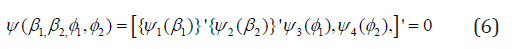In (6)are the P-dimensional vectors of the likelihood estimating equations which can be achieved by differentiating the logarithm of the likelihood function (5) with respect toand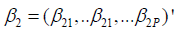respectively. The explicit expressions of The explicit expressions of 1 11 ψ (β ) and 2 21 ψ (β ) areRespectively,where Similarly, ψ31) and ψ42) can be obtained by differentiating the log-likelihood function with respect to φ1 and φ2 respectively, which has the following simplified form as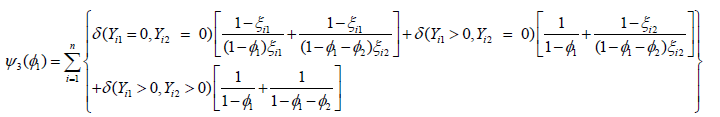AndThe estimates of the regression parameter vectors β1 and β2 and the probability parameters φ1 and φ2 can be found iteratively by using the following equationwheredenotes that the expression within the square brackets is evaluated at the rth  iteration withEstimates of φ1 φ2 and can be obtained from the logit information of the bivariate count responses vectors of Y1 and Y2 respectively. To be specific, the estimate of φ1 can be obtained from the response vector Y1 sing the logit function (logit of P(Y1=0)) ofwhere X is the covariate matrix and α is the corresponding vector of the parameters Albert et al. & Long et al. [23,24]. Similarly, the estimate of ϕ2 can be obtained from the response vector Y2 as logit of P(Y2=0). It is noteworthy to point out that in the proposed bivariate Poisson-Poisson model we only require the estimates of  and rom the logit model. Thus, in the next section we will use the estimates of φ1 and φ2

The explicit expression of the 2(P+1)×2(P+1)-dimensional information matrix in (7), is presented in the Appendix. Note that the variance-covariance matrix of the regression parameters can be calculated by taking the inverse of the information matrix in (7). This estimation techniques presented in this section are used in next section to analyze the traffic accidents data [25-30].

#### Application

In this section we apply the proposed methodology to the Virginia traffic accidents and Groningen traffic accidents data.

Virginia traffic accidents data

In this section, we apply the proposed zero-inflated Poisson-Poisson model to the Virginia traffic accidents data Leiter & Hamdan . In this data set, daily counts of accidents and corresponding fatalities were investigated by Virginia Police Department for 639 days between January 1969 and October 1970. As the number of fatalities in a given day depends on the number of accidents, we assume that the number of accidents (Y1) and the number of fatalities (Y2) follows bivariate Poisson distribution. One major concern of modelling such bivariate Poisson responses is that the data may suffer the presence of excessive zeros. For example, there may be many days with no accident occurs, thus resulting the responses to be 0. Similarly, if there is no accident then there will be no fatalities. On the other hand, there may be many accidents without any fatalities. Consequently, both responses, the number of accidents (Y1) and the number of fatalities (Y2) may be zero-innated. Thus, one needs to incorporate zero-inflated while modelling such bivariate count responses. The summary table of the Virginia traffic accident is data presented in Table 1.

Table 1: Bivariate frequency distribution of number of accidents and fatalities for Virginia traffic accidents data.Table 2: Effects of risk factors on Virginia traffic accident data under proposed bivariate zero- Inflated Poisson- Poisson model and bivariate Poisson-Poisson model without zero-inflation.aZero-inflated bivariate Poisson-Poisson regression model. bBivariate Poisson-Poisson regression model without zero-inflation.

We now use the proposed zero-inflated bivariate Poisson-Poisson model for analyzing Virginia Traffic accident data. In this data set we have only one covariate Year, which is a dummy variable with 0 if the year is 1969 and 1 if the year is 1970. We analyze the data using the zero-inflated bivariate Poisson-Poisson model (Model I) and bivariate Poisson-Poisson model without zero-inflated (Model II) and the results are presented in Table 2.

Table 2 indicates that there is no significant difference between the numbers of accidents occurs in year 1969 and 1970 under both models. Our results also indicate that the estimates of  the probability of no accident, is 0.4476. The probability of fatalities, under the condition that there are at least one accident appear to be 0.4976.

Groningen traffic accidents data

Our proposed model is also illustrated to accidents and fatalities data recorded in the city of Groningen, The Netherlands. The data were obtained from the database BRON of the Ministry of Transport, The Netherlands and presented in Table 3 of Meintanis . In particular, we have the total accidents and fatalities recorded on Sundays of each month over the period between January 1997 and December 2004 in Groningen, The Netherlands. As the number of fatalities in a given month depends on the number of accidents, we assume that the monthly number of accidents (Y1) and the number of fatalities (Y2) due to those accidents follow a bivariate Poisson distribution. Similar to the Virginia traffic accident data, we now use the proposed zero-inflated bivariate Poisson-Poisson model for analyzing Groningen traffic accident data.

To do that we consider Time and Quarter as covariates. As we have accident and fatality records of 96 months, we consider Time = 1 for January 1997, Time=2 for February 1997 … and Time = 96 for December 2004. We use logarithm of time, log(Time), as a covariate in the data analysis. The covariate Quarter was constructed by considering Quarters 1, 2, 3 and 4 as between January-March, April-June, July-September and October-December, respectively. As we have 4 categories in the covariate Quarter, we have considered three dummy variables in our analysis Quarter 2, Quarter 3 and Quarter 4 assuming Quarter 1 as the base category. It is noteworthy to point out that as in the data set monthly records of accidents were recorded, it appears that the first response variable Y1 does not have any zero responses. Thus, we assume the probability of excessive zeros, is zero to analyze Groningen traffic accidents data. Our analysis results are presented in Table 3.

Table 3 indicates that the effects of Time and various Quarters on number of accidents are similar under the proposed zero-inflated model (Model I) and the model without zero- inflation (Model 2). This is because as the number of traffic accidents (Y1) does not have any excessive zeros, the proposed zero-inflated model has behaved like the regular bivariate model. Our results in Table 3 indicates that the covariate log(Time) has negative significant effect on numbers of accidents. That means as the time increases, the numbers of accidents decreases.

Table 3: Effects of risk factors on Groningen traffic accident data under proposed bivariate zero-Inflated Poisson- Poisson model and bivariate Poisson-Poisson model without zero-inflation.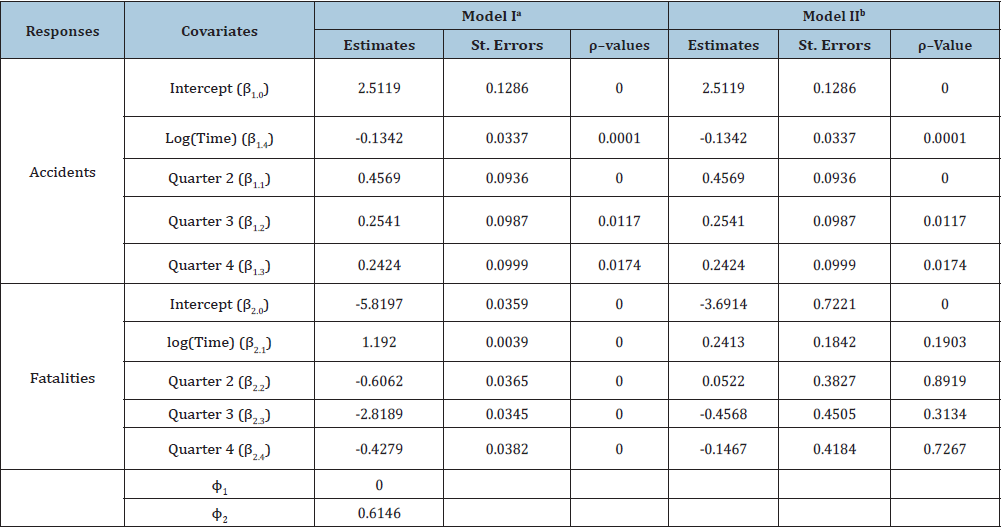aZero-inflated bivariate Poisson-Poisson regression model. bBivariate Poisson-Poisson regression model without zero-inflation.

This is probably natural as new road safety rules appear to be implemented every year to reduce the risk of accidents. Our results also indicate that the numbers of accidents are significantly higher in Quarters 2, 3 and 4 as compared to Quarter 1. This is probably because the driving activities in the other quarters are more as compared to Quarter 1 as Quarter 1 normally represents winter months including January and February be the coldest month of the year with only an average of 2 hours of sunshine every day in Groningen. Less traffic may naturally mean less traffic related accidents. Table 3 also indicates that given that at least one accident occurs, the number of fatalities is significantly lower for Quarters 2, 3 and 4 as compared to Quarter 1. This indicates that Quarter 1 has more fatalities as compared to other quarters although Quarter 1 has less traffic accidents. This is probably because although there may be less accidents in winter months (January-March) but the fatalities may be higher if accidents occur as accidents can be severe due to frost, poor visibility etc. during the winter months. Our results also indicate that given that at least one accident occurs, the number of fatalities increases as the time progresses. It is noteworthy to point out that new safety rules may reduce the number of accidents, but the number of fatalities can be increased based on severity of accidents.

In this section we have compared the analysis results of the proposed zero-inflated bi-variate Poisson- oisson model (Model I) and bivariate Poisson-Poisson model (Model II). The bivariate Poisson-Poisson model has marginal-conditional interpretations, as the bivariate marginal and conditional means of the count responses are linked to the covariates and corresponding regression parameters. It is noteworthy to point out that for the zero inflated bivariate Poisson-Poisson model, the overall mean of the count responses is smaller than the mean without the excess zero responses. This is because the overall mean under the zero-inflated model is the multiplication of the probability of non-excess zero responses and the mean of non-excess zero responses. Furthermore, in the zero-inflated model the covariate and the corresponding regression parameters are linked to the mean of the non-excess zero responses.

#### Conclusion

We propose a marginal-conditional approach based zeroinflated bivariate Poisson-Poisson model for analyzing zero-heavy bivariate count responses. Our proposed model was illustrated in two traffic accident data sets. In the traffic accident and fatalities data, the count response variables, number of accidents and number of fatalities are expected to be correlated resulting in a bivariate Poisson distribution. To analyze such bivariate data, we need to take into account the problem of excess zeros because many days and/or months may not have any occurrence of accidents and sometimes even if they have accidents, they may not any fatalities. At this backdrop, the problem of zero-inflation may distort the results obtained from the model assuming a bivariate Poisson regression model. In bivariate count data this problem poses a formidable difficulty to the users of bivariate count data in various fields of applications.

In this paper, a simple generalized linear zero-inflated model is proposed for bivariate count data using the marginal-conditional probabilities. The link functions are obtained for the bivariate zero-inflated Poisson model where the marginal probability of the number of accidents and the conditional probability of the variable on the number of fatalities for given values of the number of accidents are considered to follow the Pois- son distributions. The results indicate some notable changes both in the magnitude and direction of the estimates. Finally, the model is fitted and compared with the fitted model based on the bivariate Poisson generalized linear model. The proposed zero-inflated model shows improvement in the fitted of the model. It also displays that if the data set does not have excessive zeros, then the proposed model will likely produce the results of the bivariate Poisson models without zero-inflation. Although the proposed methodology was applied in two traffic accidents data but our approach can be generalized and utilized in the other areas of research as well.

#### Appendix

In this section we present the structure of the 2(P+1)×2(P+1)- dimensional information matrices I (γ ) as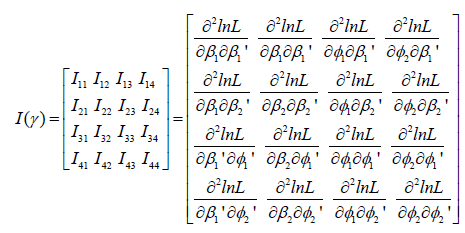After some algebra, the explicit expression of (P+1)×(P+1)- dimensional matrixcan be simplified as

Where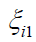is as defined in (6). Note that in I (γ ) matrix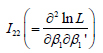is a (P+1) × (P+1) dimensional matrix with 0. Similarly, the (P+1) × 1-dimensional matrix of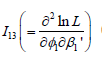can be simplified asandis a (P+1)×1- dimensional matrix with 0.

Using the derivation similar to the above, it can be shown that I23 and I24 are both (P+1)×1- dimensional matrices and have the following simplified form asThe explicit expression of the scalar quantities I33 and I34 , areandrespectively. Finally, I44 is also a scalar quantity which has the following simplified form as#### Acknowledgement

Authors acknowledge gratefully that the study is supported by the HEQEP sub-project 3293, University Grants Commission of Bangladesh and the World Bank.

We at Crimson Publishing are a group of people with a combined passion for science and research, who wants to bring to the world a unified platform where all scientific know-how is available read more...